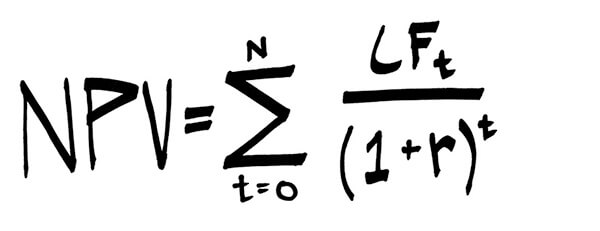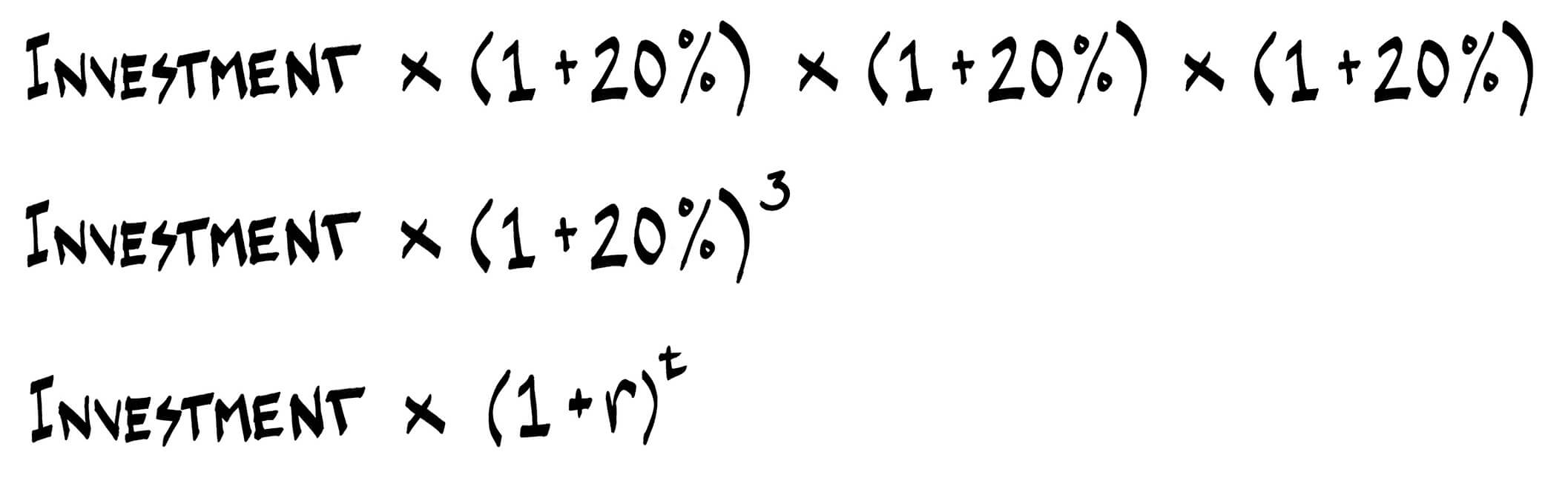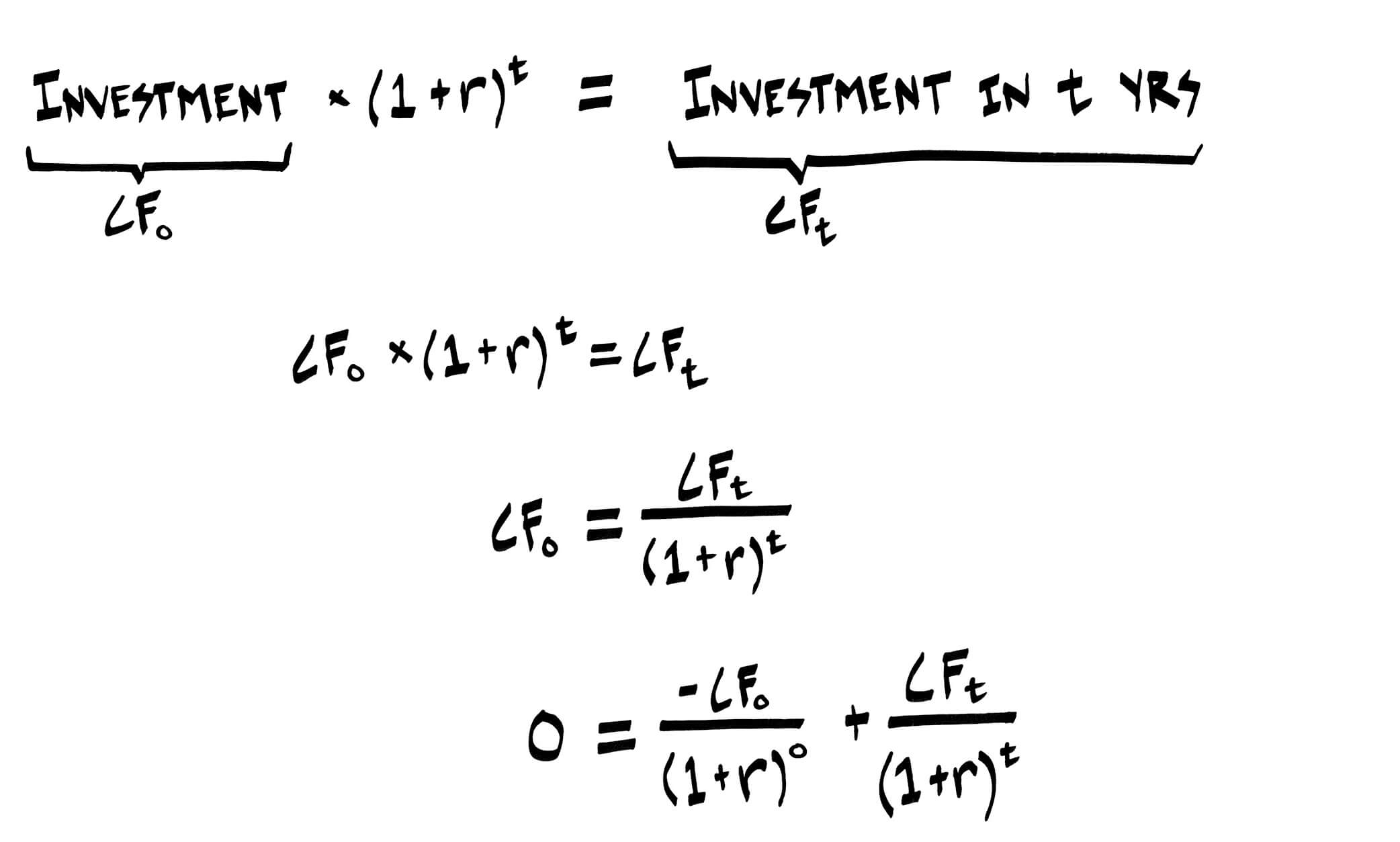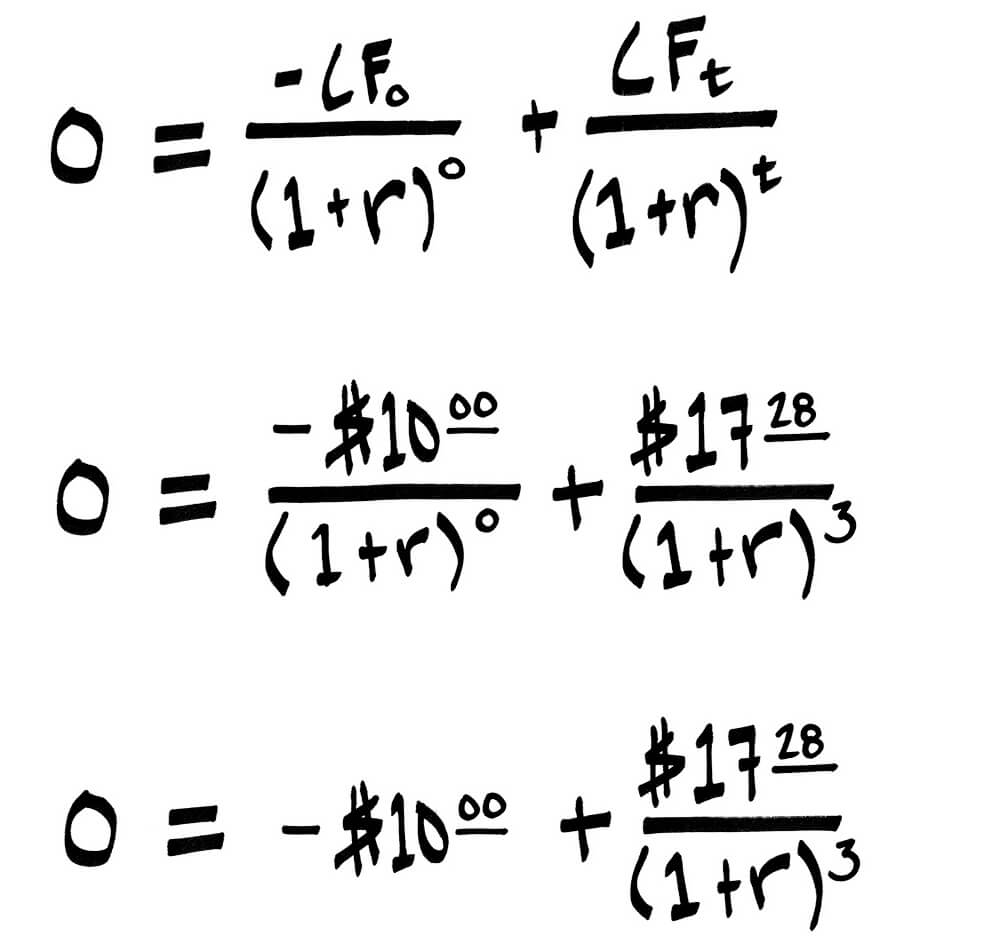Select Page

# The IRR Formula Explained

• Peter Lynch

Preface: I originally wrote this explanation of the IRR formula in response to a question asking, “Why is finance hard?”.

Even at the introductory level, finance requires so much new vocabulary that learning can feel like it involves a second language. In my opinion, it should be approached the same way.

When a student is assigned a chapter for class, the objective becomes to finish the chapter. Students read it as though they were reading a language they understand. But if every other word is new, comprehension falls. A focus on vocabulary can accelerate the process even if it feels slower to stop and digest each word.

Take the ubiquitous “IRR” as an example. A standard definition follows:

The Internal Rate of Return (“IRR”) is the rate (“r”) at which the Net Present Value (“NPV”) of all future cash inflows and outflows (“CF”) for a project is zero.

To someone new to finance, that definition means little. Worse still, the definition will likely by accompanied by an intimidating formula:To help make sense of this and demonstrate the power of really understanding new vocabulary, I created a video that explains the math by providing simple context. The math explained in the video can also be seen just below the video player.

The video explains that if a sum invested achieves an IRR of 20%, it would mean that the investment grew at a rate of 20% each year. It follows that if an investment grew by 20% each year, the value of the investment after three years could be calculated as follows:In the last step, the variable for rate “r” is substituted for 20%, and the variable for time period “t” is substituted for 3. The math should feel more approachable with familiar context. And notice that it starts to resemble the intimidating formula at the top (specifically “(1+r)^t”). Let’s take it one step further:(Note: For the last step, anything raised to the power of zero = 1, so we are dividing by 1.)

(Note 2: The last step is a combination of two steps. Step 1 is subtracting the value of “CF” in time period zero from both sides. Step 2 is matching the format of the IRR formula, which is to sum all cash inflows and outflows divided by (1+r)^t. The latter is why (1+r)^0 is included in the denominator.)

That last line is the formula that this post starts with for one cash outflow (the investment you make), and one cash inflow (the value of your investment in “t” years).

As a last step, let’s incorporate values for variables. Imagine you invested \$10 in period zero (another way of saying “today”) and three years later received \$17.28 in return. Using the formula for IRR above (where r = rate):So what you are solving for is the rate (“r”) at which the value of \$17.28 three years from now is worth \$10 today (this is Net Present Value). If you plug 20% for r in the equation above the equation will be true. Going full circle, IRR = 20%.

Focus on vocabulary and everything else will follow.